# 13 Why bodies float THRUST 13 1 Activity

• Slides: 1913. Why bodies floatTHRUST 13. 1. Activity 1)Press your thumb against a piece of paper • Now press the pointed end of a pin against the same piece of paper. • Do you notice any difference? • Answer- When pressed , the pointed end of the pin presses easily through the piece of paper. That does not happen when we press the thumb against the piece of paper.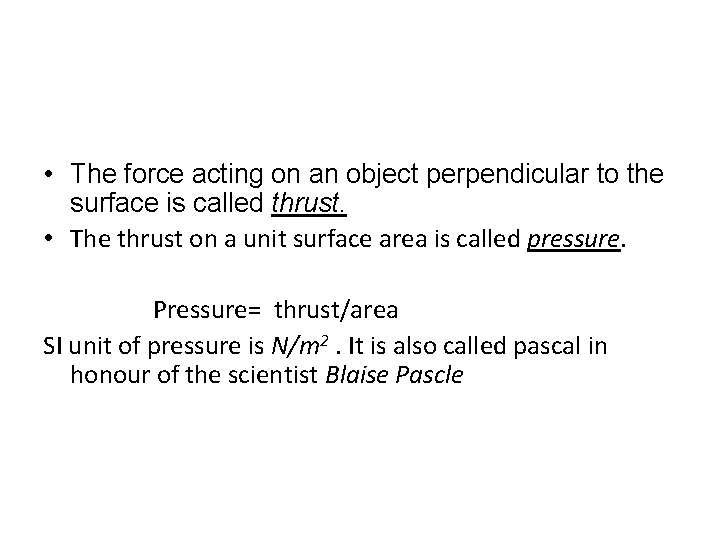• The force acting on an object perpendicular to the surface is called thrust. • The thrust on a unit surface area is called pressure. Pressure= thrust/area SI unit of pressure is N/m 2. It is also called pascal in honour of the scientist Blaise Pascle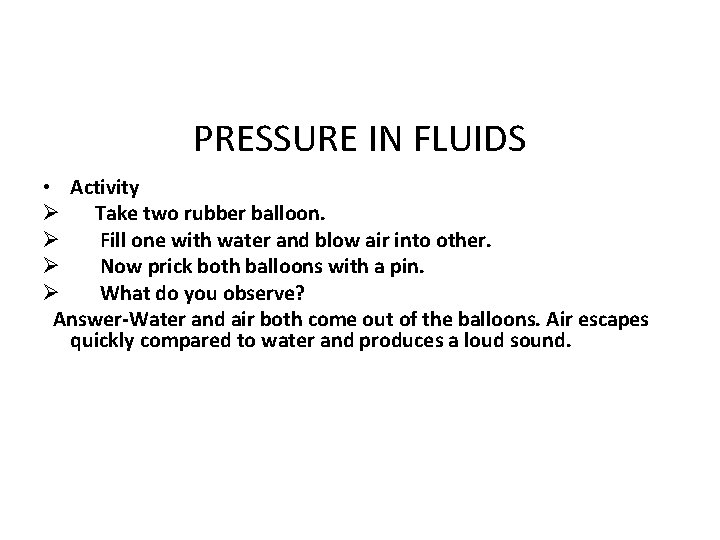PRESSURE IN FLUIDS • Activity Ø Take two rubber balloon. Ø Fill one with water and blow air into other. Ø Now prick both balloons with a pin. Ø What do you observe? Answer-Water and air both come out of the balloons. Air escapes quickly compared to water and produces a loud sound.FLUIDS • Liquids and gases are called fluids. • Fluids exert pressure on the base and walls of the container that holds them, due to weight of fluid.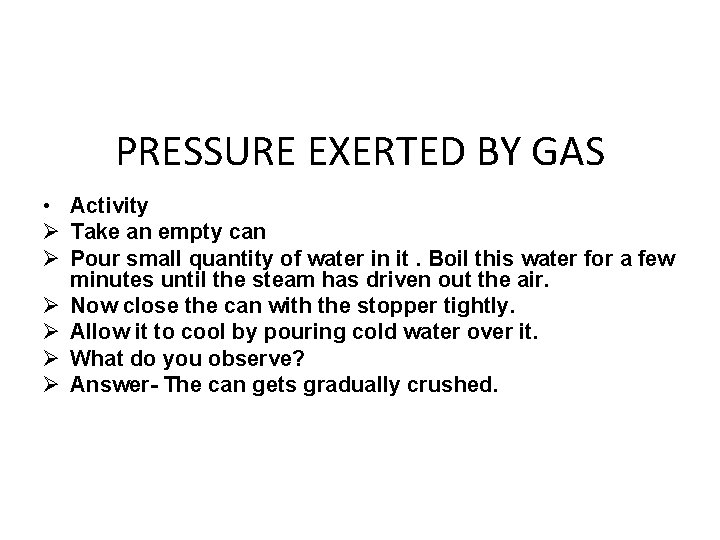PRESSURE EXERTED BY GAS • Activity Ø Take an empty can Ø Pour small quantity of water in it. Boil this water for a few minutes until the steam has driven out the air. Ø Now close the can with the stopper tightly. Ø Allow it to cool by pouring cold water over it. Ø What do you observe? Ø Answer- The can gets gradually crushed.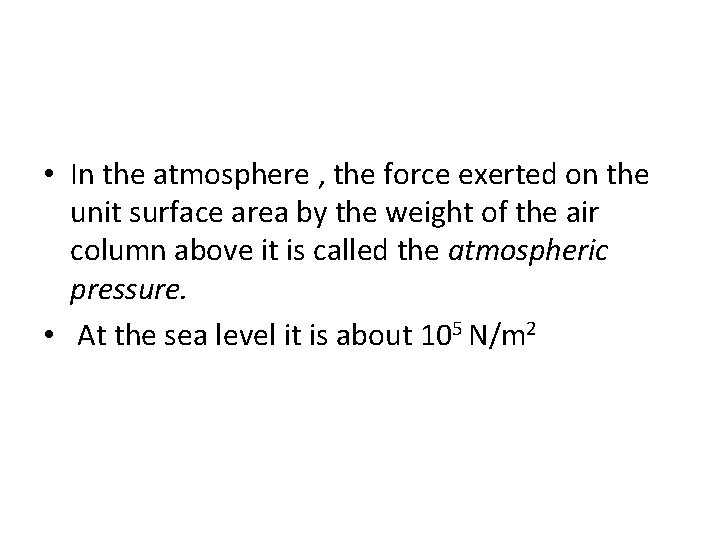• In the atmosphere , the force exerted on the unit surface area by the weight of the air column above it is called the atmospheric pressure. • At the sea level it is about 105 N/m 2LIQUID PRESSURE • Pressure increases with the depth and is proportional to the density of the liquid. • P d hBuoyant force 1] Take a full bucket of water ØTry to push inverted glass bottle into bucket. ØWhat do you experienced? ØAnswer-we experienced that an upward force on the bottle due to the water in the bucket opposing the downward motion of the bottle.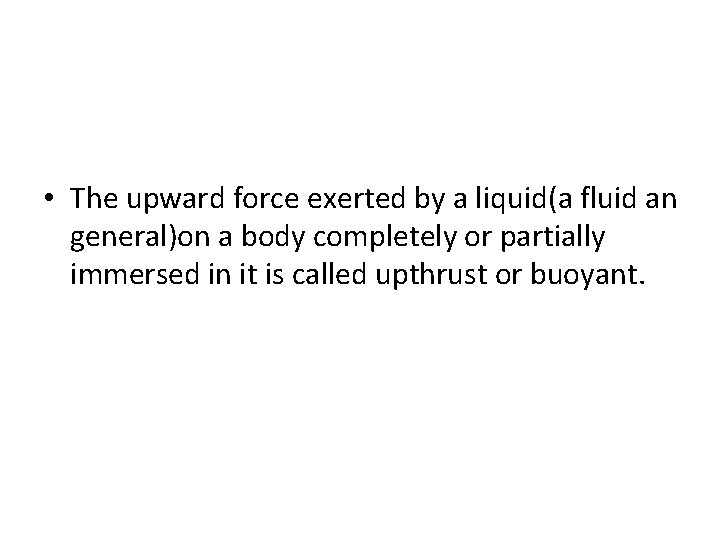• The upward force exerted by a liquid(a fluid an general)on a body completely or partially immersed in it is called upthrust or buoyant.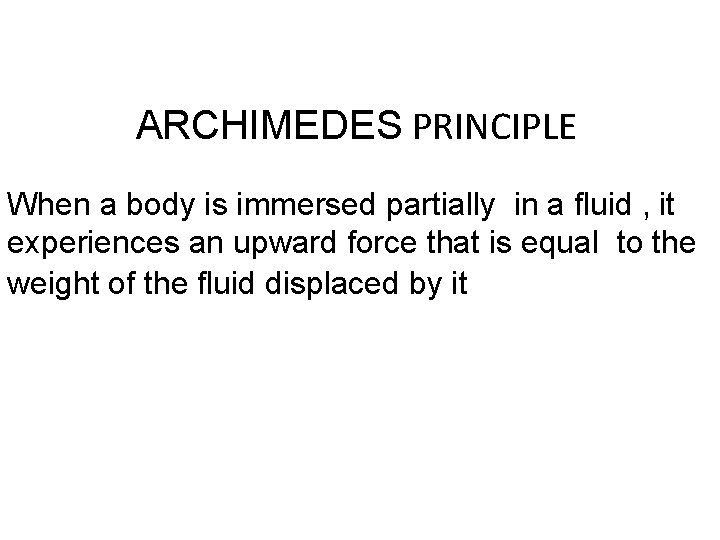ARCHIMEDES PRINCIPLE When a body is immersed partially in a fluid , it experiences an upward force that is equal to the weight of the fluid displaced by itDENSITY & RELATIVE DENSITY • Ratio of mass to volume is called density. • Density = mass / volume • SI unit is kg/m 3 and CGS UNIT is g/cm 3. • Relative density = density of substance / density of water. • It is also called specific no unit. gravity of a substance. It has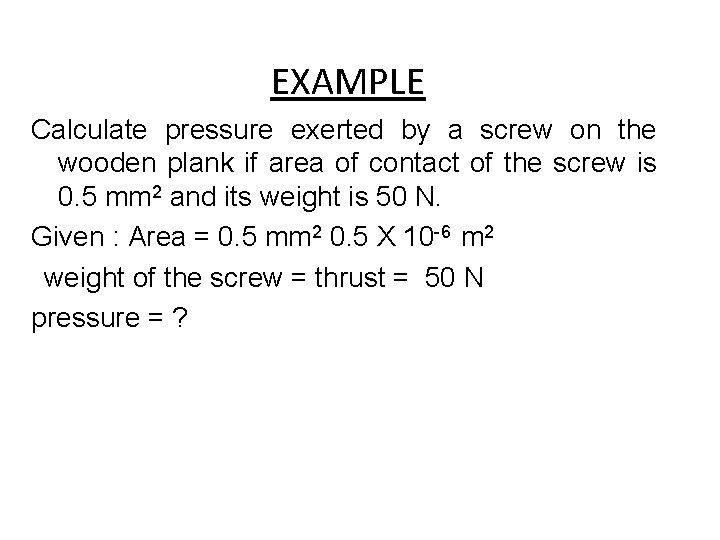EXAMPLE Calculate pressure exerted by a screw on the wooden plank if area of contact of the screw is 0. 5 mm 2 and its weight is 50 N. Given : Area = 0. 5 mm 2 0. 5 X 10 -6 m 2 weight of the screw = thrust = 50 N pressure = ?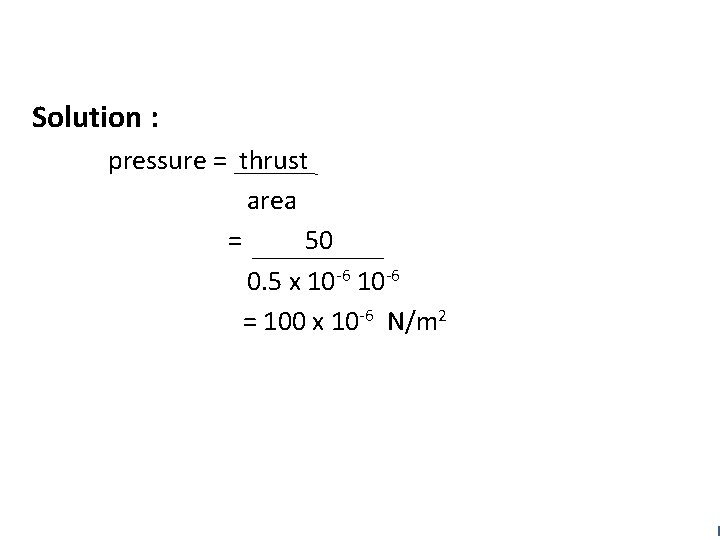Solution : pressure = thrust area = 50 0. 5 x 10 -6 = 100 x 10 -6 N/m 21) A metal block having mass 10 kg with its length 50 cm, breadth 20 cm and heigth 10 cm. Calculate pressure exerted by metal block when it is mead to lie on a table top with surface – (a) ABCD (b) DCFE (c) BCFG. Solution : Thurst = Weight of metal block = mg = 10 X 9. 8 = 98 N(a) For surface ABCD, L = 50 cm, b = 20 cm Area = length X breadth = 50 cm X 20 cm = 1000 cm 2 = 0. 1 m 2 Pressure = Thrust Area = 98 0. 1 = 980 N/m 2(b) For surface DCFE , l = 50 b = 10 cm Area = length x breadth = 50 cm x 10 cm = 500 cm 2 = 0. 05 m 2 pressure = = Thrust Area 98 0. 05 = 1960 N/m 2(c) For surface BCFG, l = 20 cm b = 10 cm Area = length x breadth = 20 cm x 10 cm = 200 cm 2 = 0. 02 m 2 Pressure = Thrust Area = 98 0. 02 = 4900 N/m 2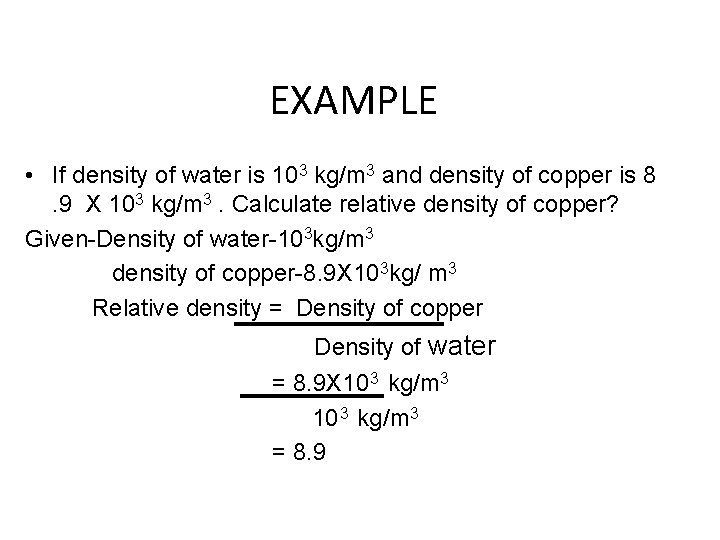EXAMPLE • If density of water is 103 kg/m 3 and density of copper is 8. 9 X 103 kg/m 3. Calculate relative density of copper? Given-Density of water-103 kg/m 3 density of copper-8. 9 X 103 kg/ m 3 Relative density = Density of copper Density of water = 8. 9 X 10 3 kg/m 3 = 8. 9